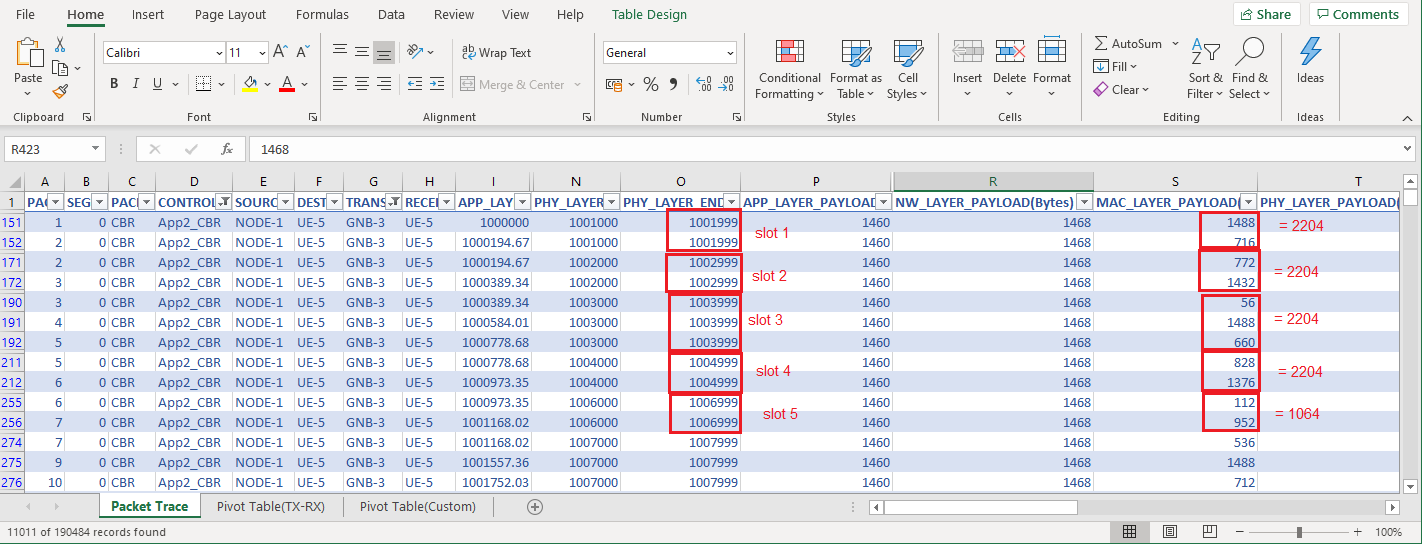Applicable Versions NetSim Standard NetSim Pro

 Applicable Releases v12.2 v13

Introduction

PRB stands for Physical Resource Block. The PRB count is determined in simulation run time by NetSim as per the inputs provided.

How to enable the PRB Log file?

Users can observe the PRB allocation and the slot allocation in the  LTENR_MAC_PRBLOG file

The PRB log file can be enabled by modifying the LTE-NR source codes as follows:

1. Goto NetSim, select Your Work(Open Simulation in v12.2)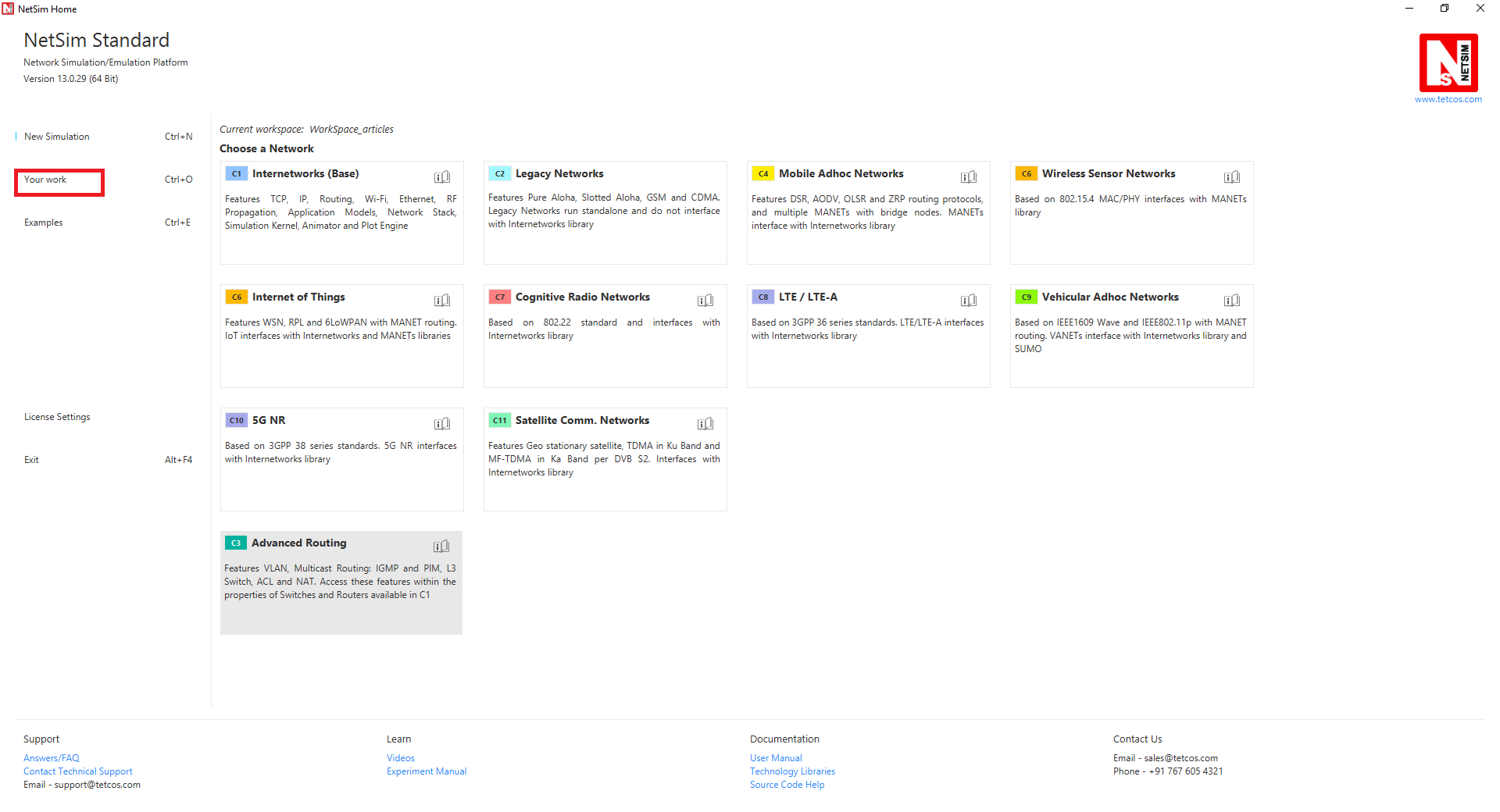2. Click on WorkSpace options3. Click on Open Code option4. Goto LTE_NR project, in the LTE_NR.c file, comment line no. #294 and uncomment line no. #295 in the get_ltenr_PRB_log_status() function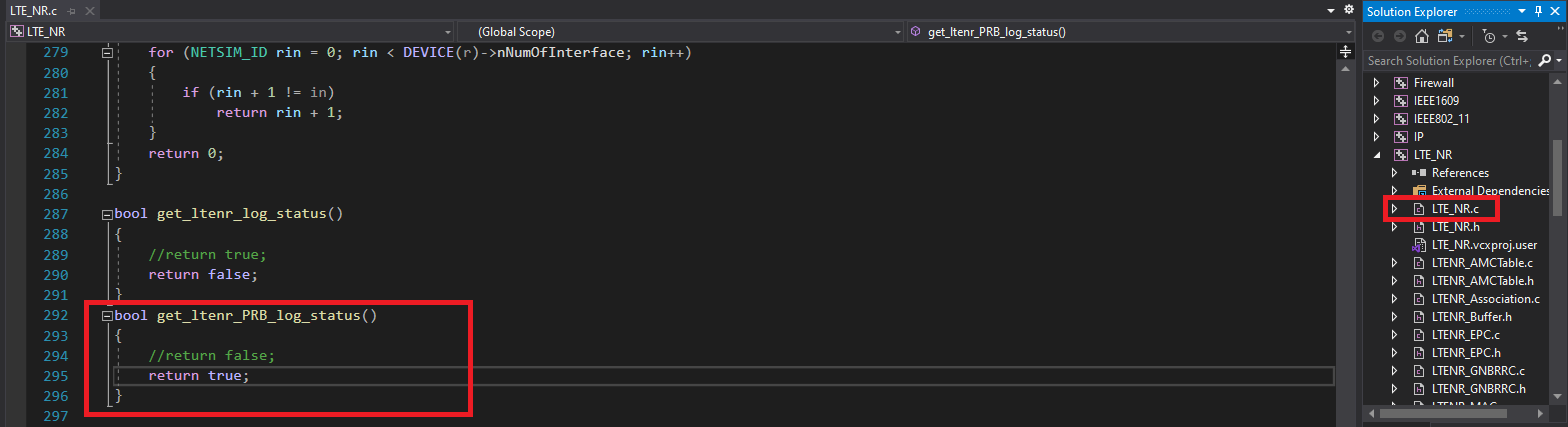5. Add the following line of code in the LTENR_PRB.c file

#define LTENR_PRB_LOG6. Right-click on LTE_NR project and click on Rebuild7. Upon successful built, the DLLs will automatically get updated in the bin directory of the current Workspace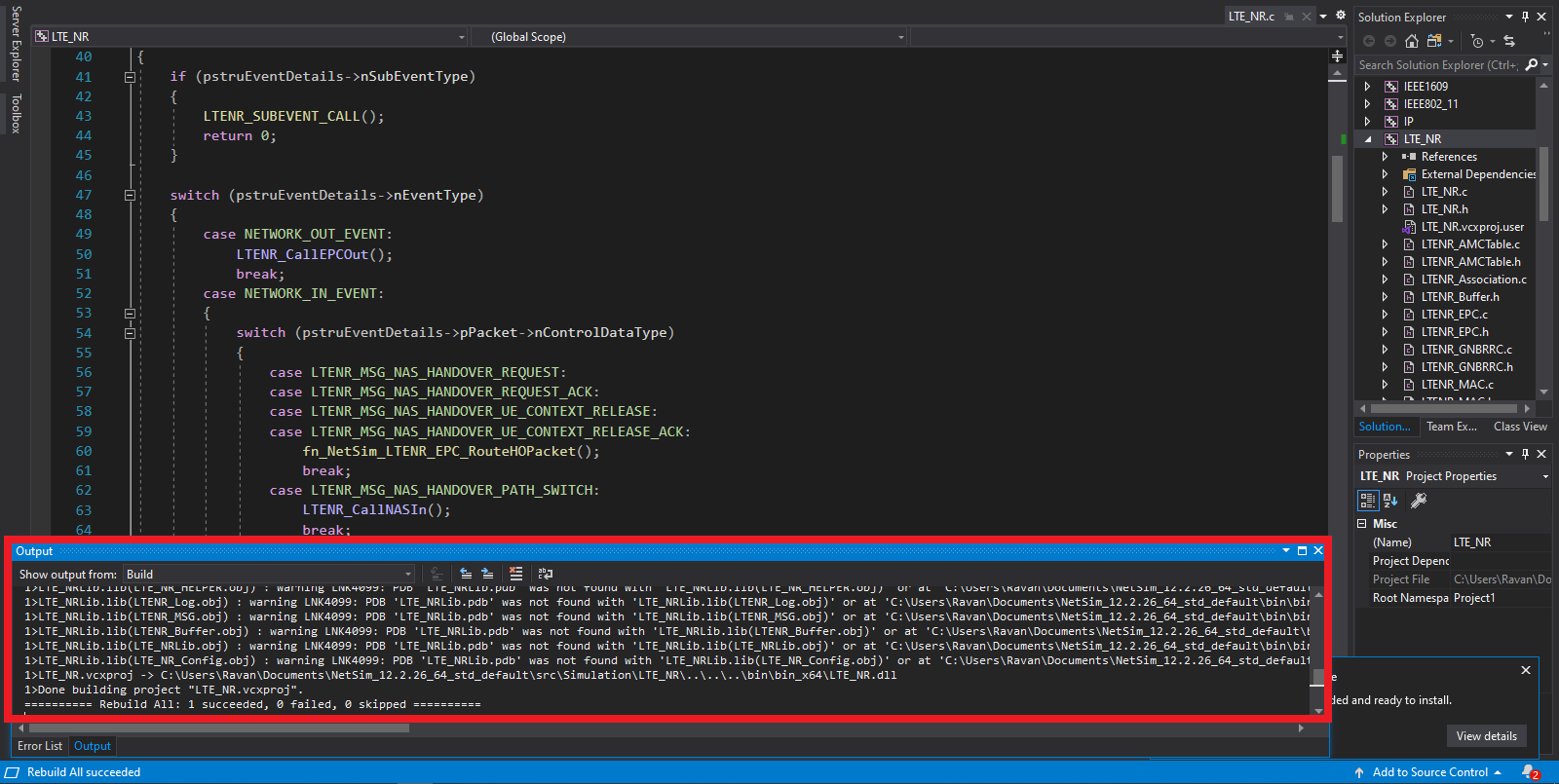This will provide the LTENR_MAC_PRBLOG.log file in the NetSim IO path.

Analyzing the PRB allocation using PRB Log file

In NetSim 5G,  slots are assigned according to the DL-UL ratio entered by the user. For example, if the ratio is 4:1, the first 4 slots will be assigned to downlink and the next slot will be assigned to uplink. This is shown below: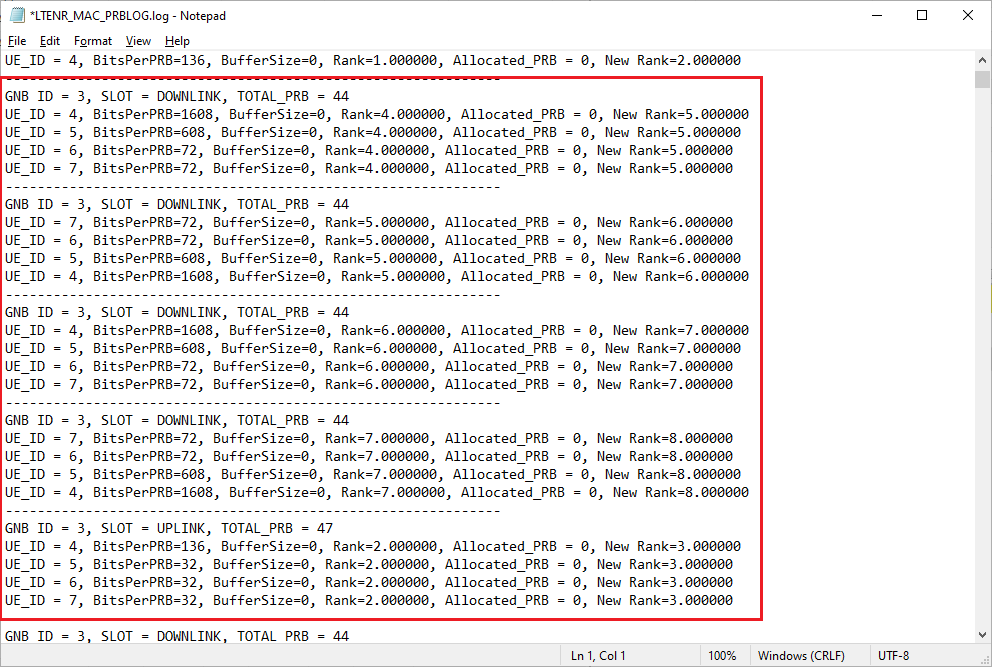In v13.0

Network scenario:

The network consists of 4UEs connected to a gNB. They are downloading 60 Mbps of data from a server which is connected to the gNB via the 5G core. The UEs are at different distances from the gNB:

UE_8 - 400m

UE_9 - 800m

UE_10 - 1200m

UE_11 - 1600m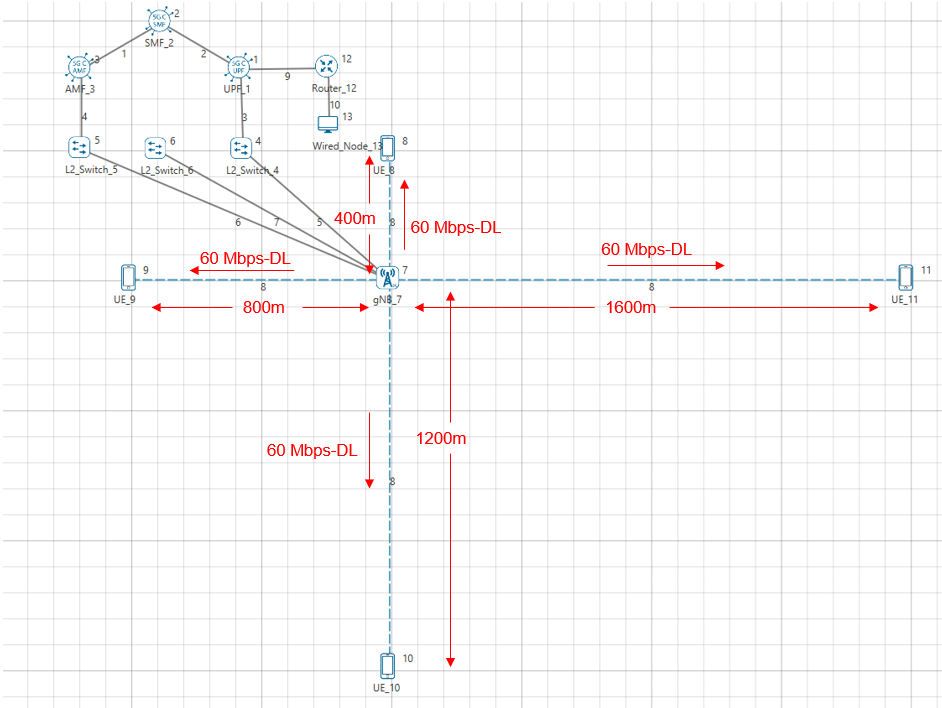Settings configured:

 Interface(5G_RAN) Properties gNB Height (m) 10 Tx_Power(dBm) 40 Tx_Antenna_Count 2 Rx_Antenna_Count 1 CA_Type Single Band CA_Configuration n78 CA_Count 1 MU 0 Channel Bandwidth (MHz) 10 PRB Count 52 MCS Table QAM64 CQI Table Table 1 X_Overhead XOH0 DL UL Ratio 4:1 Outdoor Scenario Rural Macro LOS Mode USER_DEFINED LOS Probability 0 Channel Characteristics 3GPPTR38.901-7.4.1 UE Properties Tx_Antenna_Count 1 Rx_Antenna_Count 2 Wired Link Properties Link Speed (Mbps) 100 BER 0 Propagation Delay (µs) 0 Application Properties Packet Size (Byte) 1460 Inter Arrival Time (µs) 194.67 Generation Rate (Mbps) 60 Mbps for each application Transport Control UDP Start Time (s) 1 QoS BE Simulation Parameters Simulation Time (s) 10

In v12.2

Network scenario:

The network consists of 4UEs connected to a gNB. They are downloading 60 Mbps of data from a server which is connected to the gNB via the 5G core. The UEs are at different distances from the gNB:

UE_4 - 400m

UE_5 - 800m

UE_6 - 1200m

UE_7 - 1600mSettings configured:

 Interface(LTE_NR) Properties gNB Height (m) 10 Tx_Power(dBm) 40 Downlink_MIMO_Layer_Count 2 Uplink_MIMO_Layer_Count 1 CA_Type Single Band CA_Configuration n78 CA_Count 1 MU 0 Channel Bandwidth (MHz) 10 PRB Count 52 MCS Table QAM64 CQI Table Table 1 X_Overhead XOH0 DL UL Ratio 4:1 Outdoor Scenario Rural Macro LOS Mode USER_DEFINED LOS Probability 0 Channel Characteristics Pathloss_Only Wired Link Properties Link Speed (Mbps) 100 BER 0 Propagation Delay (µs) 0 Application Properties Packet Size (Byte) 1460 Inter Arrival Time (µs) 194.67 Generation Rate (Mbps) 60 Mbps for each application Transport Control UDP Start Time (s) 1 QoS BE Simulation Parameters Simulation Time (s) 10

NOTE: A sample experiment file for the scenario explained above is attached at the end of this article, users can change the scheduling type in the Datalink Layer of gNB and then run the simulations and observe the slot allocation for different scheduling types.

PRB Allocation Logic

The bits per PRB depends on the CQI / MCS of the UE. These values can be seen from the LTENR log file (Refer section 3.14 of the NetSim 5G technology library manual to enable the LTENR log file.)

The per UE PRB allocation depends on the scheduling algorithm.

The CQI/MCS values for the various UEs at different distances as per the table shown below (CQI/MCS is noted from the LTENR logfile.)

 UE ID Distance CQI MCS UE_4 400m 14 64QAM UE_5 800m 8 16QAM UE_6 1200m 1 QPSK UE_7 1600m 2 QPSK

Case 1: Round Robin Scheduling

In the Datalink Layer of the gNB, set the Scheduling type to Round Robin and we simulate the scenario for 10s

PRB Allocation

Since the DL-UL ratio is 4:1, the first four slots will be assigned to downlink and then the next slot will be assigned to uplink.

For UE_4, the bits per PRB is 1608, for UE_5, it is 608 bits and for UE_6 and UE_7, it is 72 bits as shown in the image below.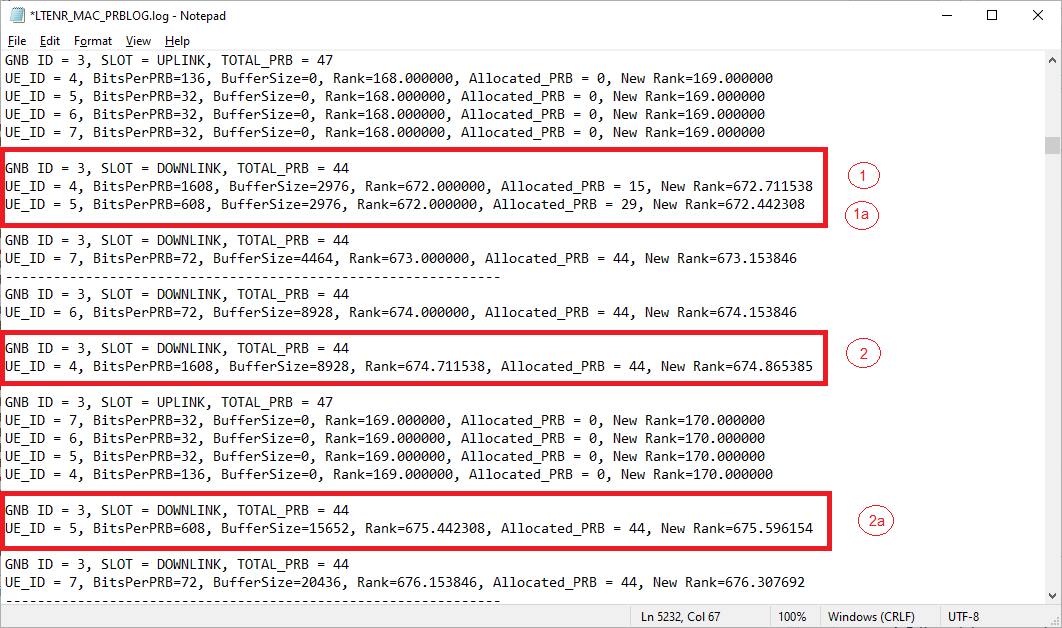The Application start time is 1s, so there are no packets to send from 0s to 1s. Hence the RLC buffer is zero during 0-1s and no PRBs are allocated.

The DL_PRB count is calculated as follows:

For the input settings, the NetSim GUI would calculate and display the PRB count as 52.

Downlink PRB count = Total PRB count - ceil of [ PRB Count (in GUI) x OH_DL] .  (Overhead is available in section 3.9 of NetSim 5G technology library manual)

= 52- ceil (52 x 0.14) = 52 - ceil (7.28) = 52 - 8 = 44

The total PRB count is 44.  In this, the allocated PRB for UE_4 is 15 and the remaining 29 is allocated to UE_5.

The reason for the allocation of 15 PRBs to UE_4 is explained below:

Consider UE_4,

Buffer size = 2,976 bytes

Bits per PRB = 1,608

Total PRB = 44

PRBs required to transmit the data in buffer equals [2,976 * 8 / 1608]= 15 PRBs (therefore Allocated PRB = 15)

Bits per slot = bits per PRB x allocated PRB = 15 x 1608 = 24,120 bits = 3,015 bytes

The slot can transmit a maximum of 3,015 bytes.

Since the PHY transmits the packets in the buffer, it will allocate 2976 bytes to the first slot.

In the next slot, the total PRB count is 44 and all the PRBs are allocated to UE_4 since the bytes in the RLC buffer is 8,928

Allocated PRB= 44 for UE_4,

Buffer Size = 8,928 bytes

Bits per slot=bits per PRB x allocated PRB=  44 x 1608 = 70,752 bits = 8,844 Bytes

Since the slot can handle a maximum of 8,844 bytes, the packet is fragmented.  The 8844 bytes consist of five packets of size 1488 bytes. The 6th packet s fragmented; 1st fragment is 1404B and the 2nd fragment is 84B. The same is shown in the image below.

In the Packet Trace, filter the control packet type to App_1_CBR (since App_1_CBR is configured in UE_4), the Transmitter_Id to gNB since the application configured is the downlink. The MAC Layer Payload shows bytes (of packets in a slot.In the same way, for UE_5,

The capacity of a slot (bits) is calculated as explained below:

Buffer size = 2,976 bytes

Bits per PRB = 608

Total PRB = 44

PRBs required to transmit the data in buffer equals [2,976 * 8 / 608]= 39

Allocated PRB =29, since the total PRB count is 44 and 15 PRBs are allocated to UE_4

Bits per slot = bits per PRB x allocated PRB = 29 x 608 = 17,632 bits = 2,204 bytes

The slot can transmit a maximum of 2,204 bytes.

The PRB first allocates the packets in the buffer, it will allocate, say 2,204 bytes to the first slot.

Since the slot can transmit a maximum of  2,204 bytes, the packet is fragmented. The first fragment is 716 bytes and the second fragment is 772 bytes. The 2,204 bytes consists of one packet of size 1488 bytes and one packet fragment of size 716 bytes.

In the next slot, the total PRB count is 44 and all the PRBs are allocated to UE_5 since the bytes in the RLC buffer is 15,652

Allocated PRB= 44 for UE_5,

Buffer Size = 15,652 bytes

Bits per slot=bits per PRB x allocated PRB=  44 x  608 = 26,752 bits = 3,344 Bytes

Since the slot can transmit a maximum of 3,344 bytes.  The 3,344 bytes consist of one packet of size 772 bytes (a fragment of a packet fragmented in the previous slot), one packet of size 1488 bytes, and one packet fragment of size 1084 bytes.

The same is shown in the image below.

In the Packet Trace, filter the control packet type to App_2_CBR (since App_2_CBR is configured in UE_5), the Transmitter_Id to gNB since the application configured is the downlink. The MAC Layer Payload shows bytes of packets in a slot.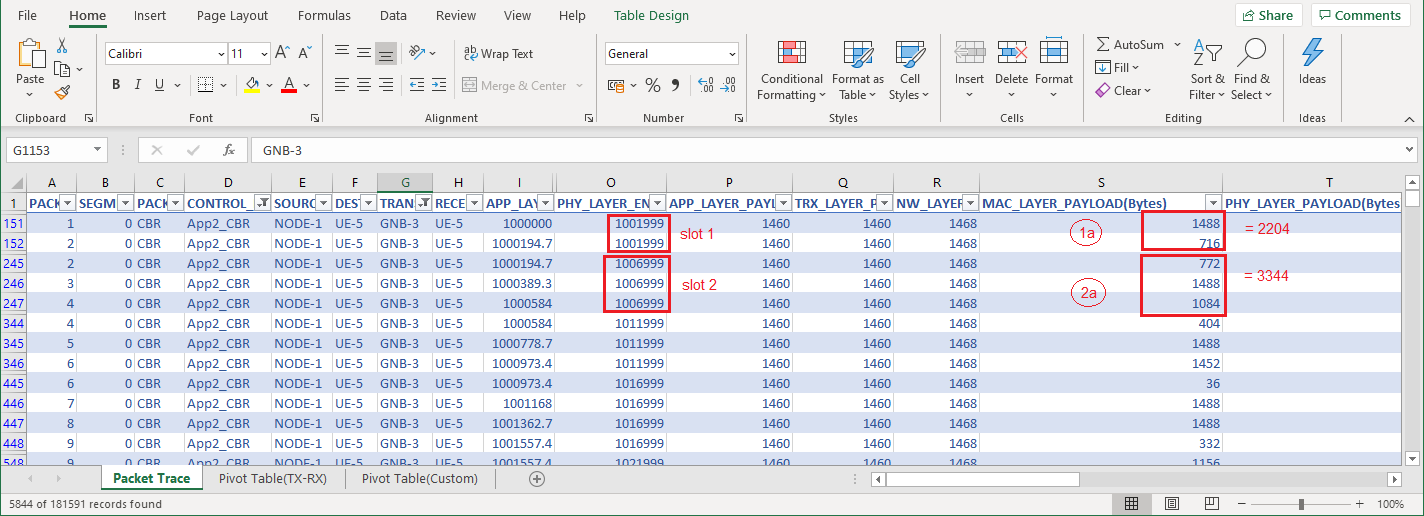Case 2: Proportional Fair Scheduling

In the Datalink Layer of the gNB, set the Scheduling type to Proportional Fair and simulate the scenario for 10s

PRB Allocation

Since the DL-UL ratio is 4:1, the first four slots will be assigned to downlink and then the next slot will be assigned to uplink.

For UE_4, the bits per PRB are 1608 bits, for UE_5, it is 608 bits and for UE_6 and UE_7, it is 72 bits as shown below.The Application start time is 1s, so there are no packets to send from zeroth second to first second, Hence the RLC buffer is zero during 0-1s and no PRBs are allocated.

The reason for the allocation of 15 PRBs to UE_4 is explained below:

Consider UE_4,

Buffer size = 2,976 bytes

Bits per PRB = 1,608

Total PRB = 44

PRBs required to transmit the data in buffer equals [2,976 * 8 / 1608]= 15 (therefore, Allocated PRB = 15)

Bits per slot = bits per PRB x allocated PRB = 15 x 1608 = 24,120 bits = 3,015 bytes

The slot can handle a maximum of 3,015 bytes.

Since the PHY transmits the packets in the buffer, it will allocate 2976 bytes to the first slot.

Similarly, the next three slots will be having packets of 2976 bytes as the allocated PRB is 15.

In the fifth slot, the total PRB count is 30 and all the PRBs are allocated to UE_4 since the bytes in the RLC buffer is 5,952 bytes.

Allocated PRB= 30 for UE_4,

Buffer Size = 5,952 bytes

Bits per slot=bits per PRB x allocated PRB=  30 x  1608 = 48,240 bits = 6,030 bytes

Since the slot can handle a maximum of 6030 bytes, the fifth slot is allocated with 5952 bytes data from the buffer.

The same is shown in the image below.

In the Packet Trace, filter the control packet type to App_1_CBR (since App_1_CBR is configured in UE_4), the Transmitter_Id to gNB since the application configured is the downlink. The MAC Layer Payload shows bytes of packets in a slot.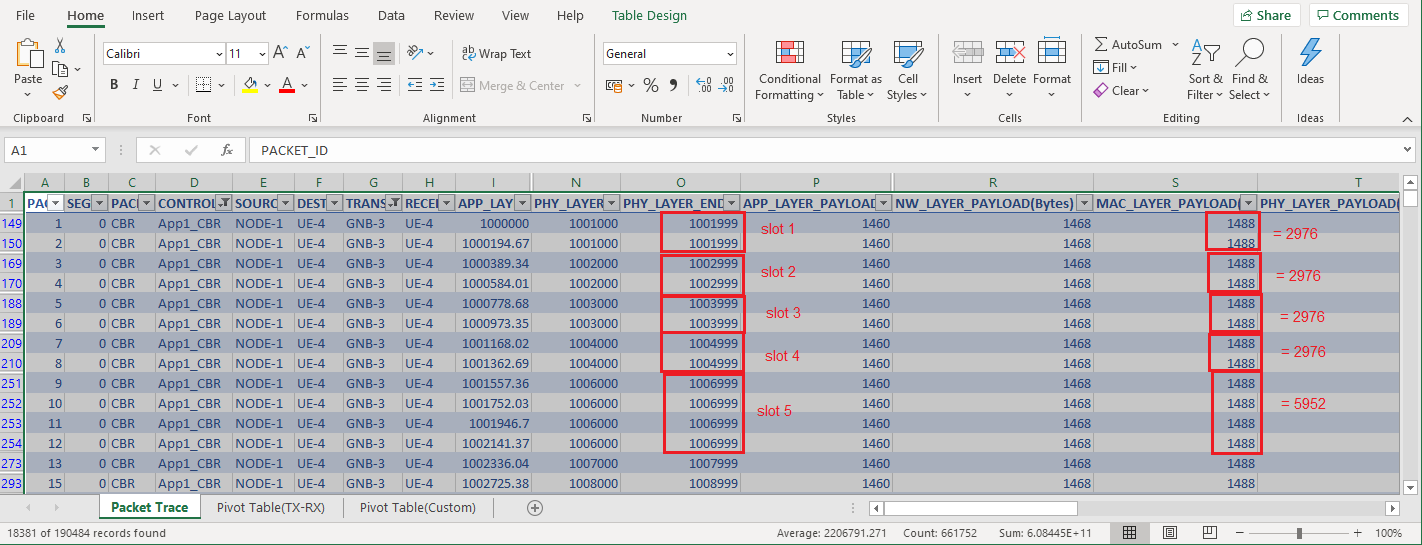In the same way, for UE_5,

The capacity of a slot (bits) is calculated as explained below:

Buffer size = 2,976 bytes

Bits per PRB = 608

Total PRB = 44

PRBs required to transmit the data in buffer equals [2,976 * 8 / 608]= 39

Allocated PRB =29, since the total PRB count is 44 and 15 PRBs are allocated to UE_4

Bits per slot = bits per PRB x allocated PRB = 29 x 608 = 17,632 bits = 2,204 bytes

The slot can handle a maximum of 2,204 bytes to a slot.

The PHY transmits the packets in the buffer, it will allocate 2976 bytes to the first slot.

Since the slot can handle a maximum of  2,204 bytes. The 2,204 bytes consists of one packet of size 1488 bytes and one packet fragment of size 716 bytes.

Similarly, the next three slots will be having packets of 2204 bytes as the allocated PRB is 29.

In the fifth slot, the total PRB count is 44 and 14 PRBs are allocated to UE_5 since the bytes in the RLC buffer is 7552

Allocated PRB= 14 for UE_5,

Buffer Size = 7552 bytes

Bits per slot=bits per PRB x allocated PRB=  14 x  608 = 8,512 bits = 1,064 Bytes

The 1,064 bytes consist of one packet of size 112 bytes, and one packet fragment of size 952 bytes. The same is shown in the image below.

In the Packet Trace, filter the control packet type to App_2_CBR (since App_2_CBR is configured in UE_5), the Transmitter_Id to gNB since the application configured is the downlink. The MAC Layer Payload shows bytes of packets in a slot.